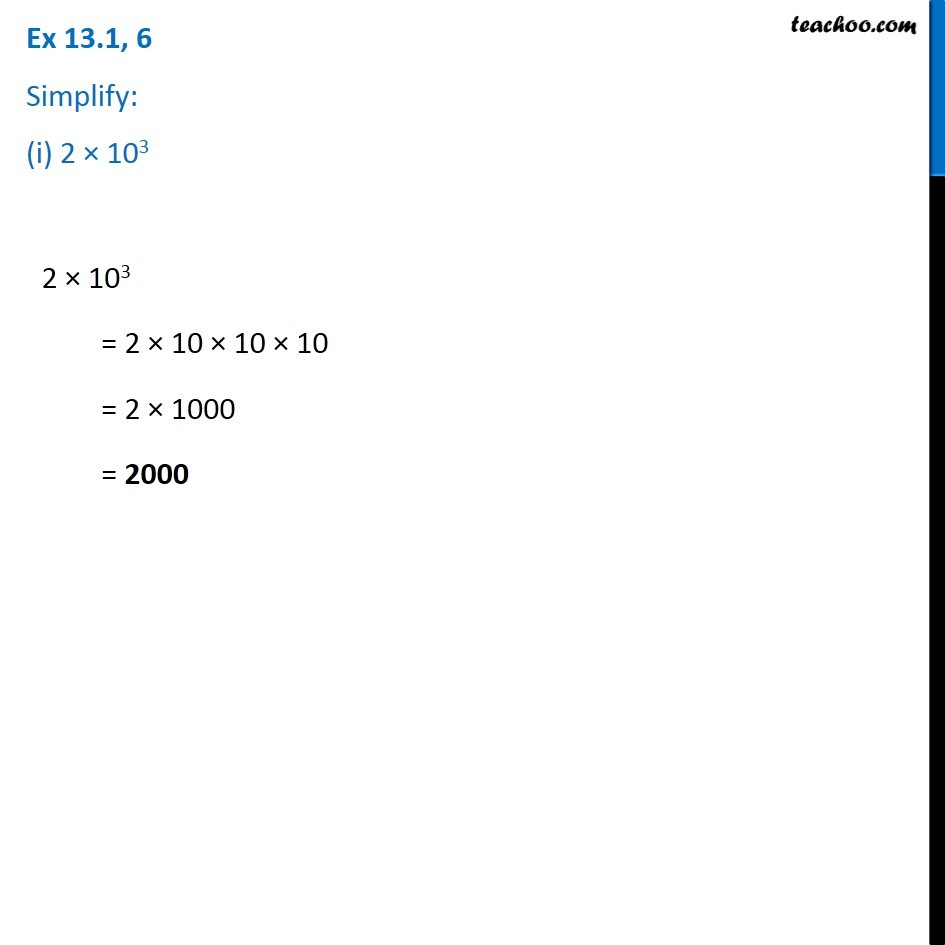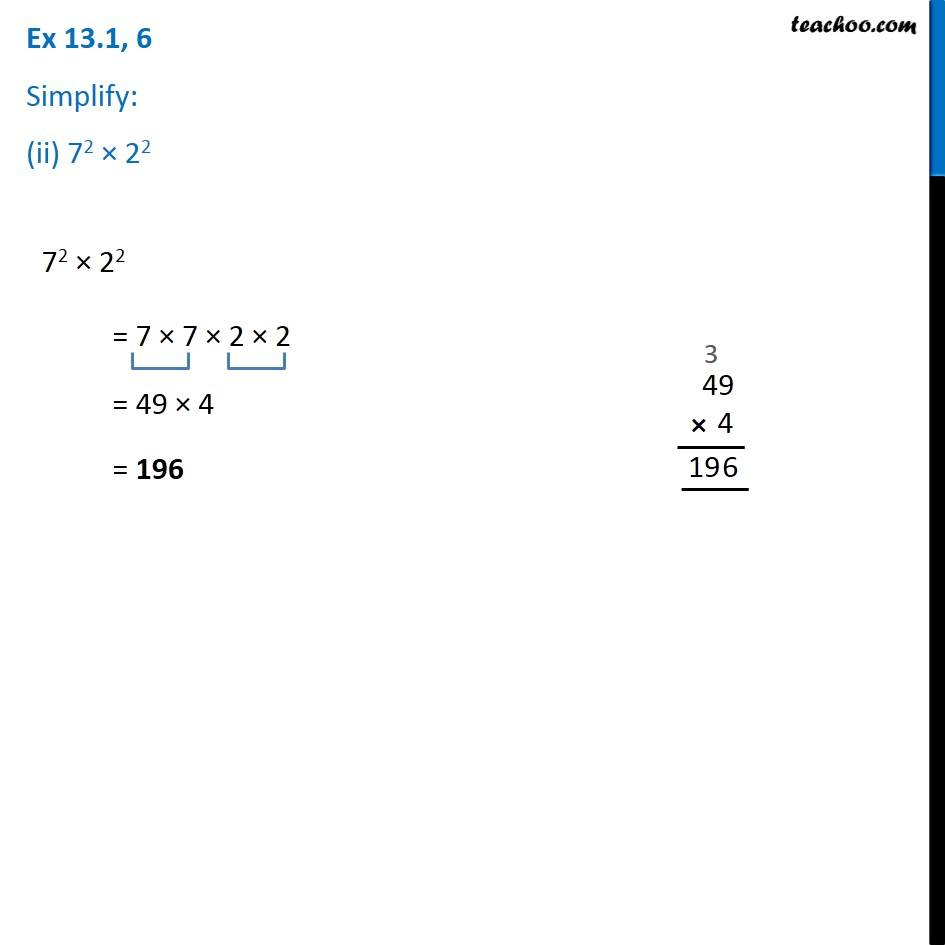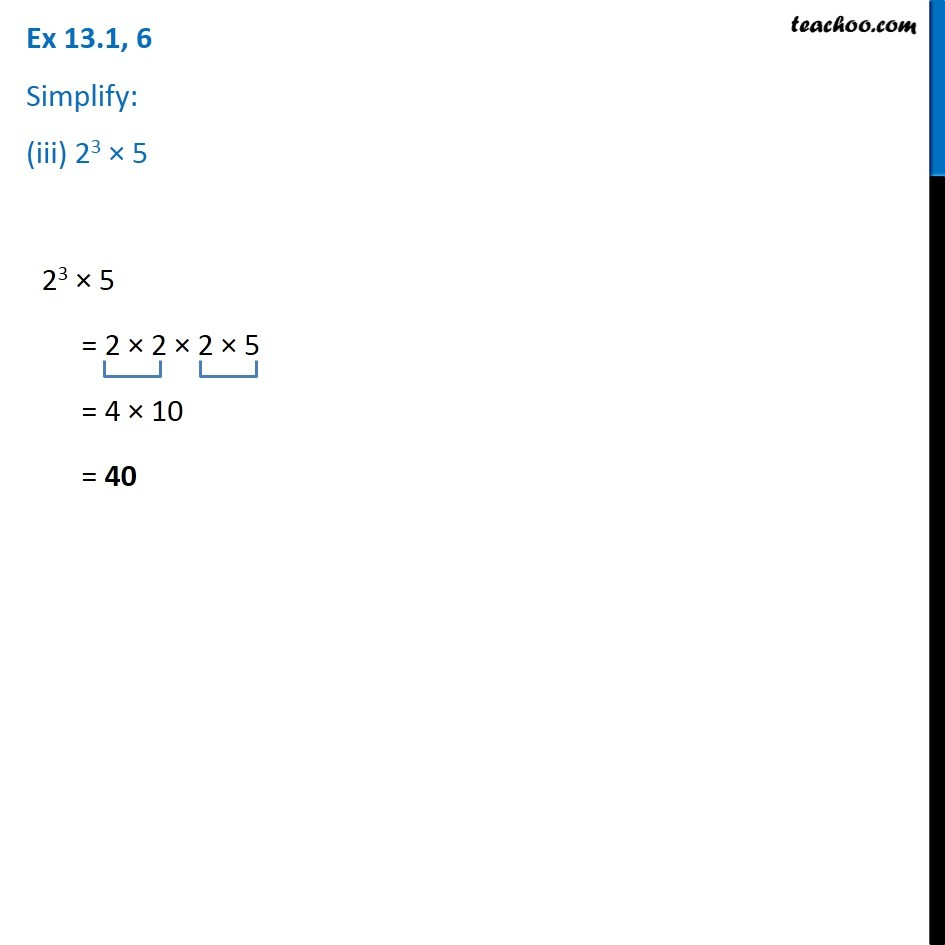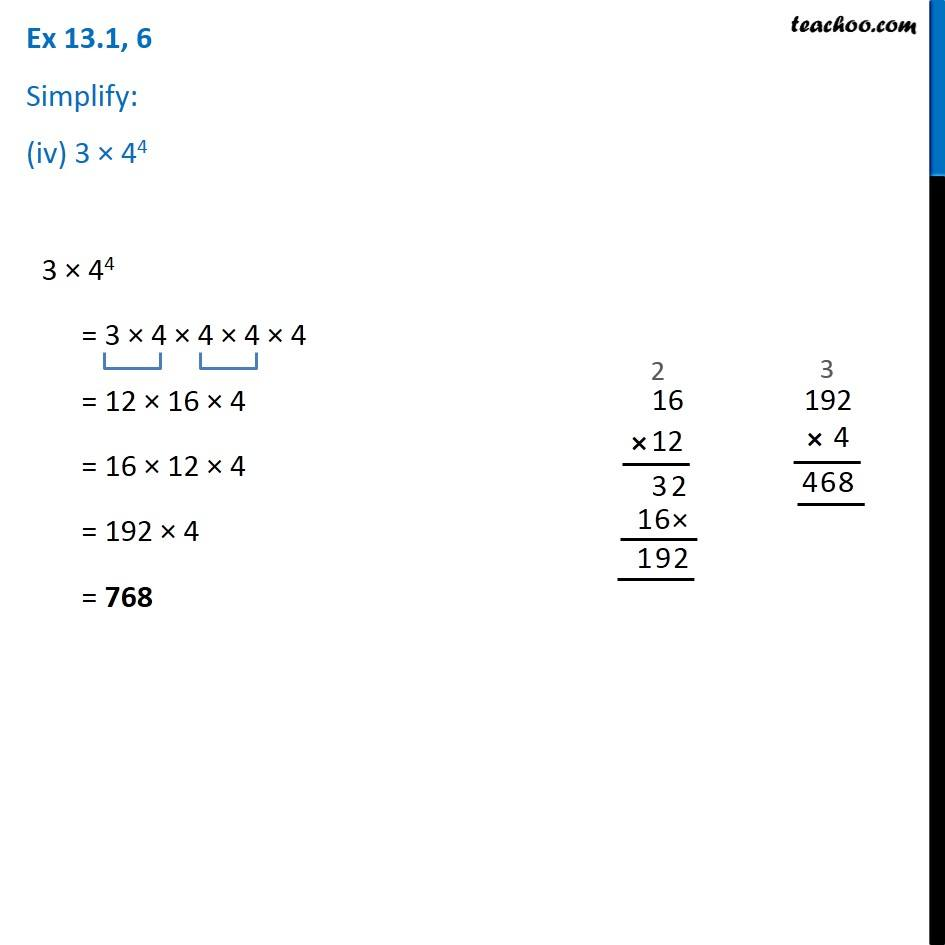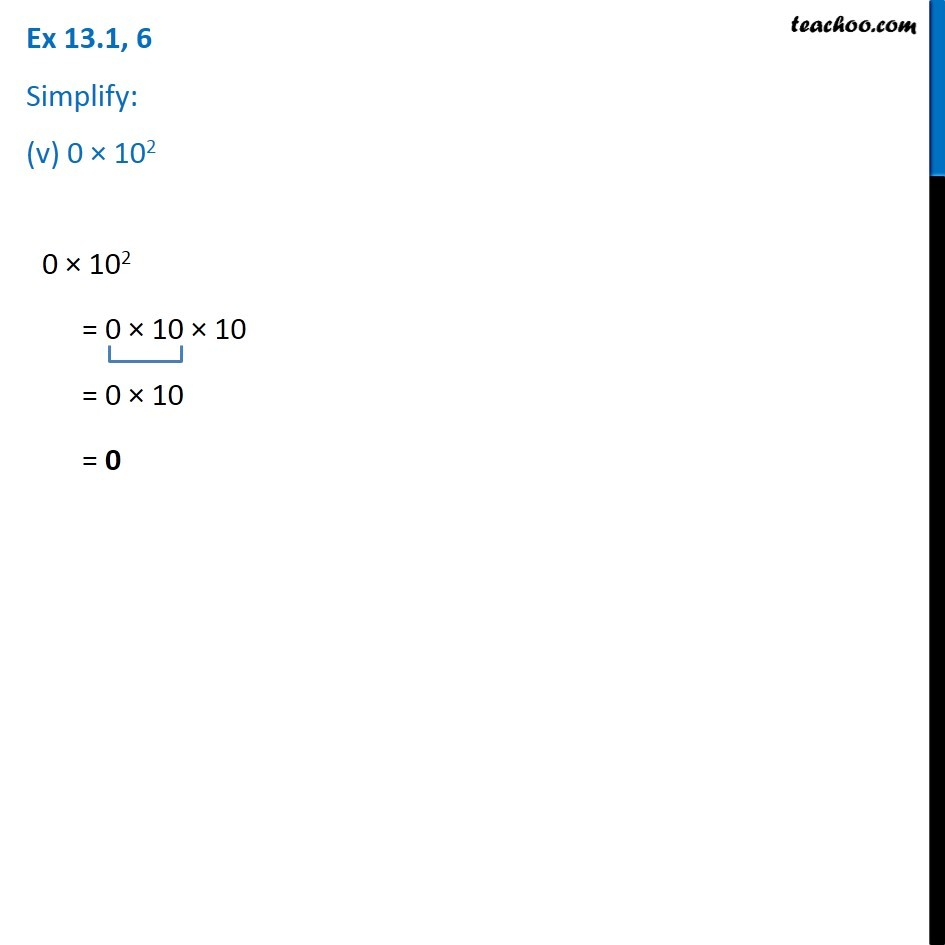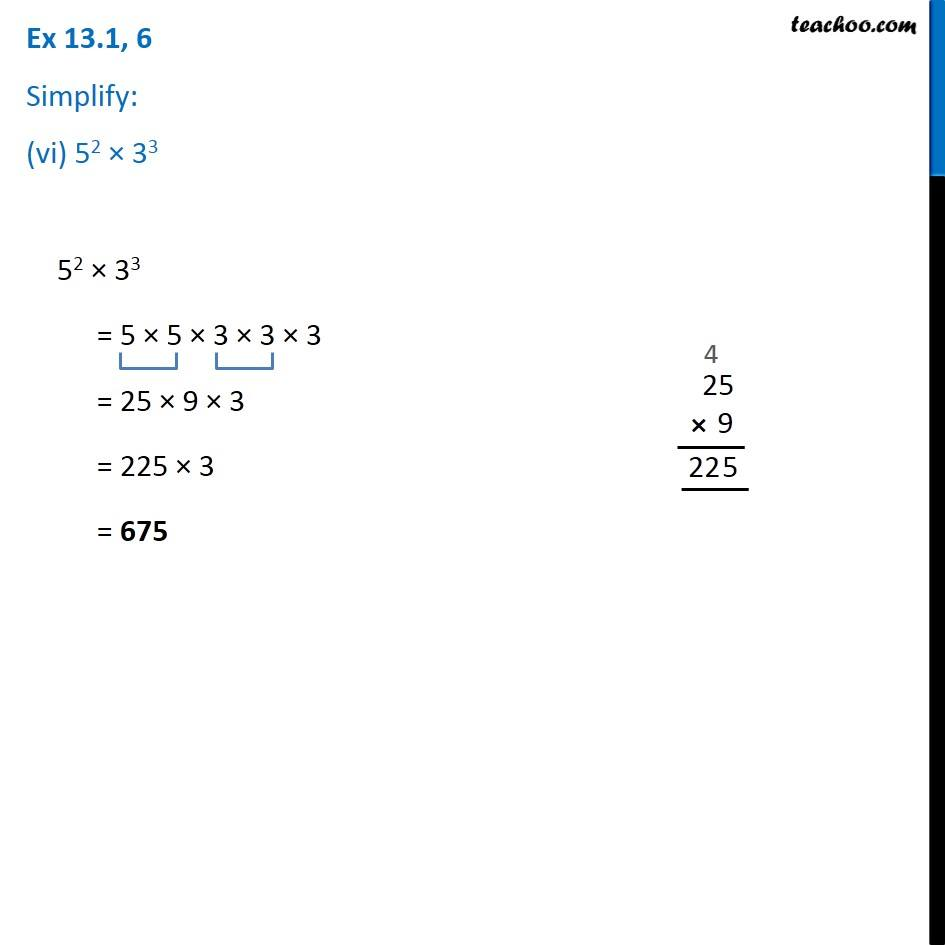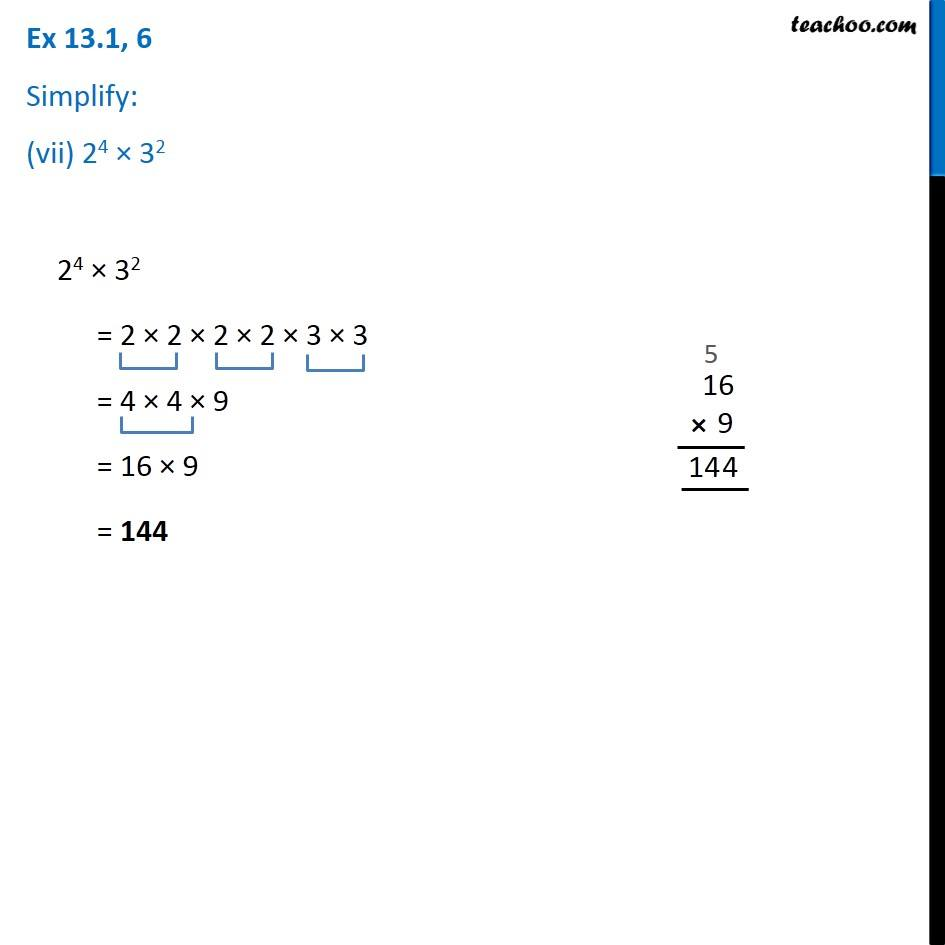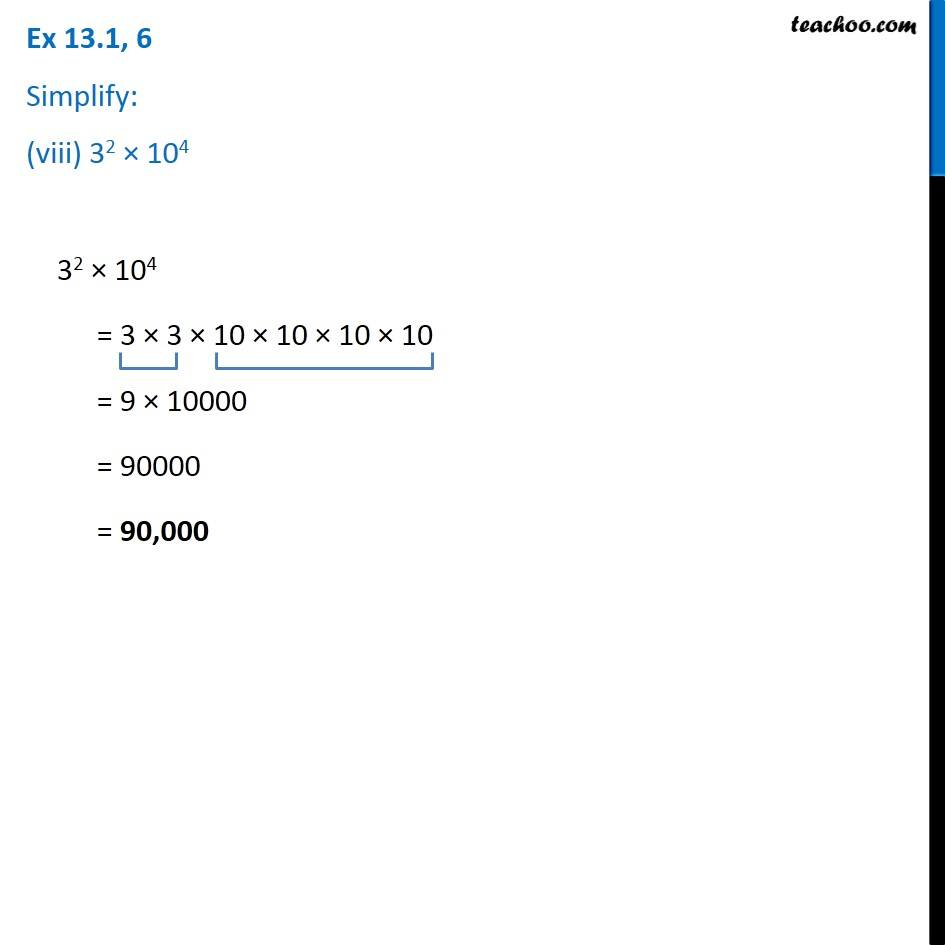1. Chapter 13 Class 7 Exponents and Powers
2. Serial order wise
3. Ex 13.1

Transcript

Ex 13.1, 6 Simplify: (i) 2 × 103 2 × 103 = 2 × 10 × 10 × 10 = 2 × 1000 = 2000 Ex 13.1, 6 Simplify: (ii) 72 × 22 72 × 22 = 7 × 7 × 2 × 2 = 49 × 4 = 196 Ex 13.1, 6 Simplify: (iii) 23 × 5 23 × 5 = 2 × 2 × 2 × 5 = 4 × 10 = 40 Ex 13.1, 6 Simplify: (iv) 3 × 44 3 × 44 = 3 × 4 × 4 × 4 × 4 = 12 × 16 × 4 = 16 × 12 × 4 = 192 × 4 = 768 Ex 13.1, 6 Simplify: (v) 0 × 102 0 × 102 = 0 × 10 × 10 = 0 × 10 = 0 Ex 13.1, 6 Simplify: (vi) 52 × 33 52 × 33 = 5 × 5 × 3 × 3 × 3 = 25 × 9 × 3 = 225 × 3 = 675 Ex 13.1, 6 Simplify: (vii) 24 × 32 24 × 32 = 2 × 2 × 2 × 2 × 3 × 3 = 4 × 4 × 9 = 16 × 9 = 144 Ex 13.1, 6 Simplify: (viii) 32 × 104 32 × 104 = 3 × 3 × 10 × 10 × 10 × 10 = 9 × 10000 = 90000 = 90,000

Ex 13.1

Chapter 13 Class 7 Exponents and Powers
Serial order wise